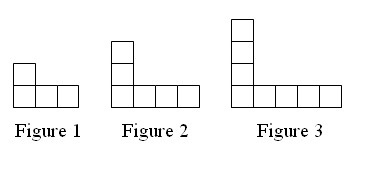### Home > AC > Chapter 3 > Lesson 3.2.2 > Problem3-83

3-83.

Draw Figure 0 and Figure 4 for the pattern below on graph paper. 3-83 HW eTool (Desmos).1. Represent the number of tiles in each figure with:

• An $x→y$ table.

• An algebraic rule.

• A graph.

Try and find a connection between the number of the Figure and the number of tiles.

2. Without drawing Figure 5, predict where its point would lie on the graph. Justify your prediction.

Use the information found in part (a), and find what $y$ is when $x=5$ on the graph.

Complete the table in the eTool below.
Click the link at right for the full version of the eTool: AC 3-84 HW eTool